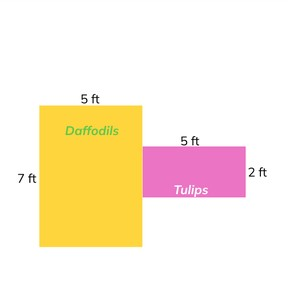Calculating area of combined shapes

# Calculating area of combined shapes

I can calculate the area of combined shapes.

No account needed.8,000 schools use Gynzy92,000 teachers use Gynzy1,600,000 students use Gynzy

## General

Students will review the basics of finding area with formulas and parallel lines. Students will extend this knowledge by calculating the area of combined shapes. A worksheet is provided so that students can follow along and work with the slides.

## Standards

CCSS.Math.Content.4.MD.A.3

## Learning objective

Students will be able to calculate the area of combined shapes.

## Introduction

Students will review the concept of parallel lines. They will trace parallel lines found in everyday objects. This is important because they will use parallel lines to find missing measurements.

## Instruction

Students begin by reviewing the formulas for the area of a square and rectangle. They are then presented with a combined shape on a grid. They count the squares to find the area. Next, students are shown the shape with measurements and they learn that you can find the area of each shape using the formulas, and then add to find the combined area. They practice this skill on the following slide. Students then move on to working with the outline of combined shapes. They are shown that you begin by dividing the shape, then finding the length and width of each of the shapes. They may find colored pencils helpful when working with these shapes. The next step is to find the area of each shape, and then proceed to add them. Continuing on, students use parallel lines to find a missing measurement. They practice finding missing measurements on outline shapes and then follow the steps to find the combined area. There is also a challenge slide to find the area of 3 combined shapes. A final exercise requires the students to find the combined area to solve a story problem about gardening.

## Quiz

Students respond to ten multiple-choice and true/false questions.

## Closing

Students are given several shapes to drag to a grid and find their combined area. They assign a unit of measurement to the combined shape. They are also encouraged to draw their own squares or rectangles with the toolbar if they are able.

## Instruction materials

Colored pencils or markers to trace around each shape of the combined shapes

### The online teaching platform for interactive whiteboards and displays in schools

• Save time building lessons

• Manage the classroom more efficiently

• Increase student engagement